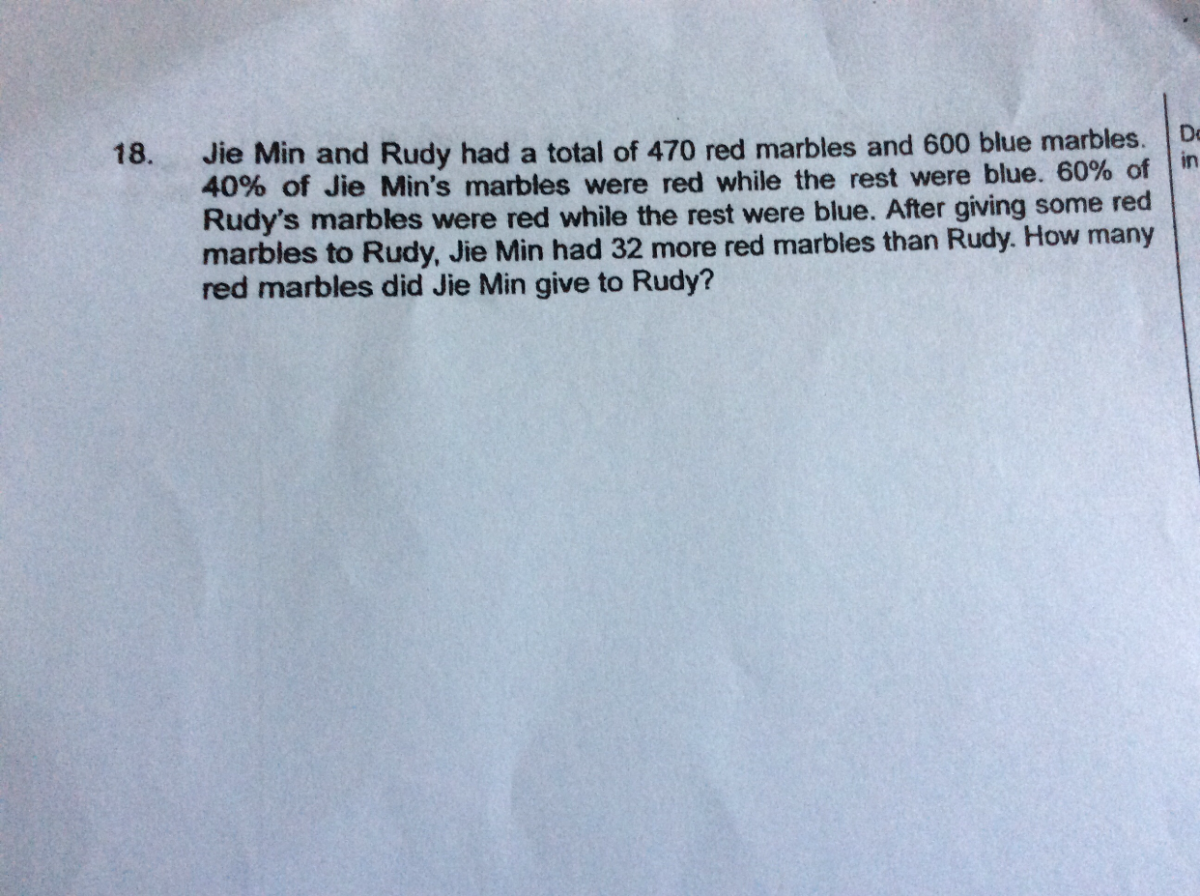# QuestionJie Min
red : 4u
blue : 6u

Rudy
red : 6p
blue : 4p

4u + 6p ——- 470
16u + 24p ——-1880
6u + 4p ——– 600
36u + 24p ——- 3600
36u – 16u = 20u ——- 3600 – 1880 = 1720
1u ——- 1720/20 = 86
86 x 4 = 344 (red marbles Jie Min had at first)
4 x 86 + 6p = 344 + 6p ——- 470
1p ——- (470 – 344)/6 = 21
6p ——- 6 x 21 = 126 (red marbles Rudy had at first)
470 – 32 = 438
438/2 = 219 (red marbles Rudy had in the end)
219 – 126 = 93

Ans : 93 red marbles.

0 Replies 1 Like ✔Accepted Answer